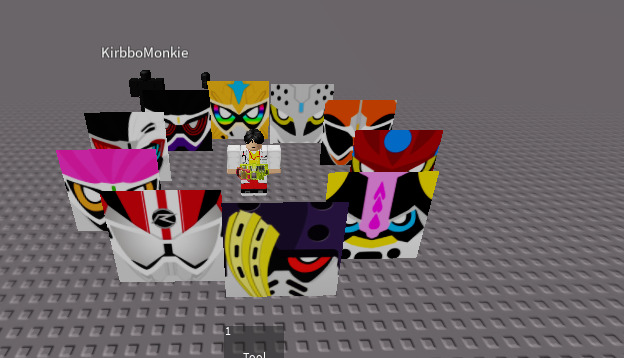# Make spinning parts stop on a certain one

So basically, I have this script here that has spinning parts to spin around a character, and it should land on a specific one, but the script just spins and stops at random. How do I make it stop on the one with orange eyes and pink hair?

``````local character = workspace:WaitForChild("CleetusSama"):WaitForChild("HumanoidRootPart") -- test code to try out

local DISTANCE = 10 -- radius/distance to rotate from the player.

local increment = 0

local PartClone = game.ReplicatedStorage.CParts:Clone()

PartClone.Parent = game.Workspace

local parts = PartClone:GetChildren()

local offset = 360/#parts -- make a perfect angle distance for each part no matter how many parts by dividing a whole circle by the amount of parts.

local rs = game:GetService("RunService")

rs.Heartbeat:Connect(function(dt)
increment += dt * (math.abs(math.sin(tick() * 1)) * 125)
print(increment)
for i,v in pairs(parts) do -- v is the current part
local trueOffset = offset * i
v.Position = Vector3.new(character.Position.X + math.sin(math.rad(increment+trueOffset)) * DISTANCE,character.Position.Y ,character.Position.Z + math.cos(math.rad(increment+trueOffset)) * DISTANCE)
end
end)

``````It looks like this

so, are there no answers for this?